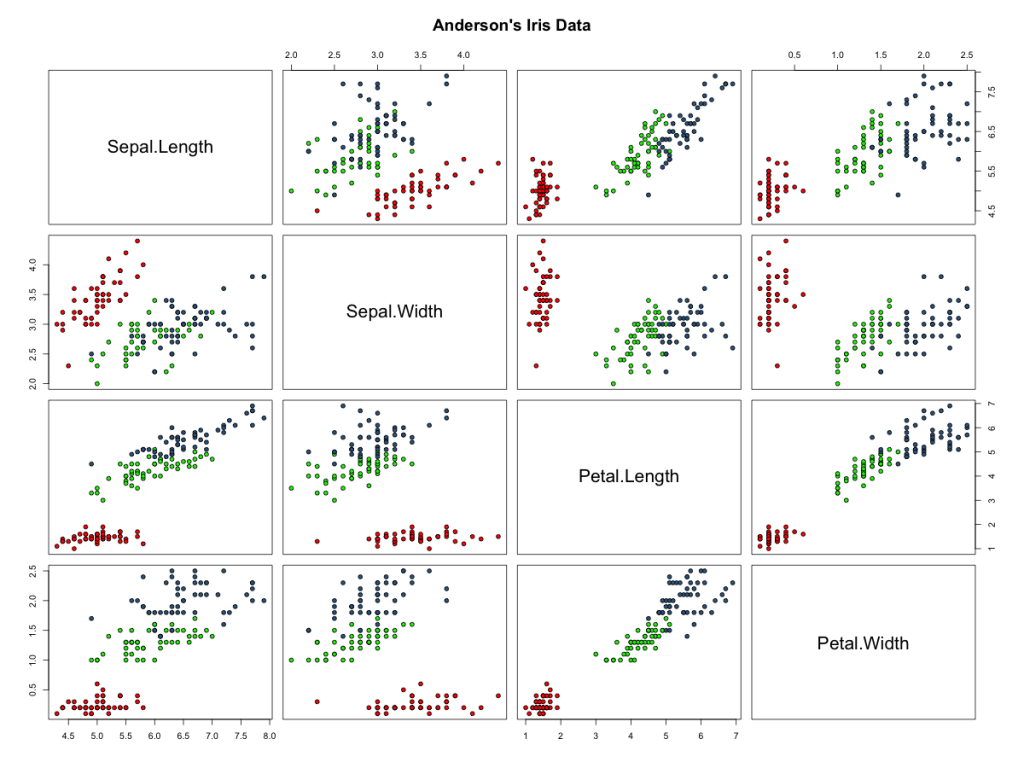# Correlation Plots in R

The standard function for correlation plots in R is pairs(), which generates a matrix of scatter plots based on all pairwise combinations of variables in a data object.  The standard graph looks something like this after a little color enhancement:”Click to enlarge

The code behind this plot is simple:

Meanwhile, new package extensions for R have made better use of the graphical systems intrinsic to R.  One of those packages is ggplot2.  Another closely related package is GGally, which makes use of multi-layerd graph layouts to extend the basic correlation plot in R.  The alternative approach relies on the function ggpairs() to allow users to control what information appears where in a matrix of plots.  As a result, a range of text and graph types can now be blended into the correlation plot layout.  For example:

The code is a little more demanding, but still relatively simple, and the resulting graph is much more informative:

This entry was posted in Data Science, ggplot2, R Graphics, R Programming. Bookmark the permalink.